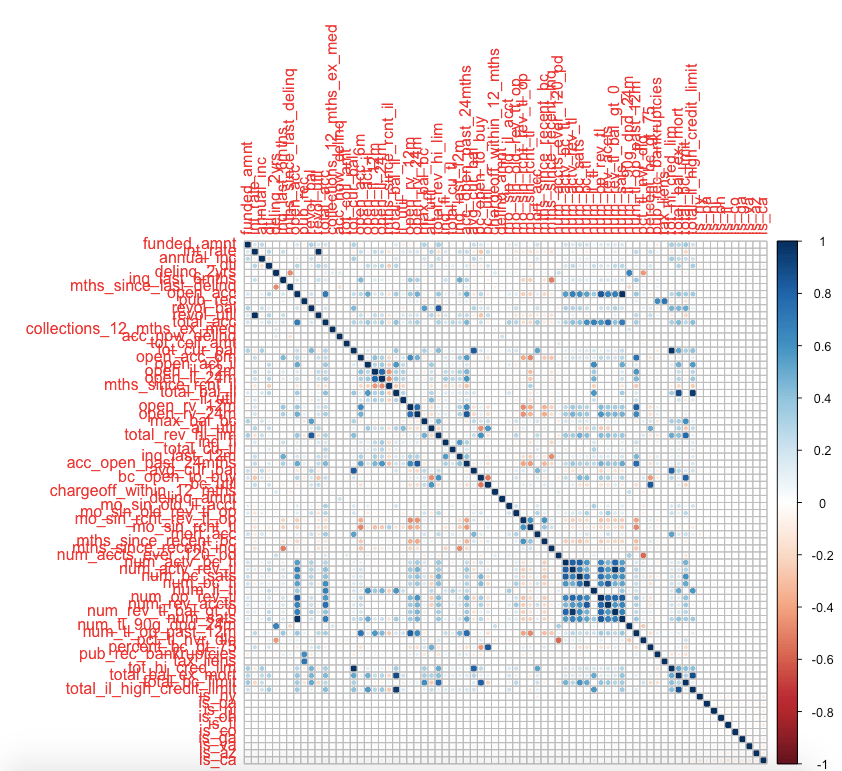# Data Cleaning in R - Part 3

### Default by States

We take a look at default rate for each state. We filter out states that have too small number of loans(less than 1000):

tmp = data_train %>% filter(loan_status=="Default") %>% group_by(addr_state) %>% summarise(default_count = n())
tmp2 = data_train %>% group_by(addr_state) %>% summarise(count = n())
tmp3 = tmp2 %>% left_join(tmp) %>% mutate(default_rate = default_count/count)

> tmp3
# A tibble: 50 x 4

1 AK           112            42        0.375
2 AL           557           194        0.348
3 AR           315           122        0.387
4 AZ          1090           316        0.290
5 CA          6173          1837        0.298
6 CO           952           222        0.233
7 CT           563           147        0.261
8 DC            86            14        0.163
9 DE           118            31        0.263
10 FL          3014           933        0.310
# ... with 40 more rows
>


### Order States by Default Rate

We can order states by default rate to identify states with highest and lowest default rates.

#order by highest default rate

high_default = (tmp3 %>% filter(count > 1000) %>% arrange(desc(default_rate)))[1:10,"addr_state"]$addr_state high_default  "NY" "PA" "NJ" "OH" "FL" "IL" "NC" "MI" "TX" "CA"  # order by lowest default rate low_default = (tmp3 %>% filter(count > 1000) %>% arrange((default_rate)))[1:10,"addr_state"]$addr_state

> low_default
 "CO" "GA" "VA" "AZ" "CA" "TX" "MI" "NC" "IL" "FL"
>


We then create binary variable for 5 highest states and 5 lowest states discard the rest.

data_train$is_ny = ifelse(data_train$addr_state=="NY",1,0)
data_train$is_pa = ifelse(data_train$addr_state=="PA",1,0)
data_train$is_nj = ifelse(data_train$addr_state=="NJ",1,0)
data_train$is_oh = ifelse(data_train$addr_state=="OH",1,0)
data_train$is_fl = ifelse(data_train$addr_state=="FL",1,0)

data_train$is_co = ifelse(data_train$addr_state=="CO",1,0)
data_train$is_ga = ifelse(data_train$addr_state=="GA",1,0)
data_train$is_va = ifelse(data_train$addr_state=="VA",1,0)
data_train$is_az = ifelse(data_train$addr_state=="AZ",1,0)
data_train$is_ca = ifelse(data_train$addr_state=="CA",1,0)

data_train\$addr_state = NULL

rm(tmp, tmp2, tmp3, high_default, low_default)


### Remove Correlated Features

We will investigate if there are any correlation among features.

corrplot(cor(data_train[getNumericColumns(data_train)],use="na.or.complete”))We found some features are quite correlated, we can remove correlated features with findCorrelation function. The function will find all correlated pairs that have correlation exceed a specified threshold and try to remove one of them in such a way that overall correlation is reduced.

> high_corr <- findCorrelation(cor(data_train[getNumericColumns(data_train)]), cutoff = .75)
> high_corr = getNumericColumns(data_train)[high_corr]
> high_corr
 "open_acc"                   "num_sats"                   "total_acc"
 "acc_open_past_24mths"       "num_op_rev_tl"              "num_rev_accts"
 "num_bc_sats"                "num_tl_op_past_12m"         "total_rev_hi_lim"
 "total_bc_limit"             "total_bal_ex_mort"          "num_actv_rev_tl"
 "num_rev_tl_bal_gt_0"        "open_rv_24m"                "tot_hi_cred_lim"
 "tot_cur_bal"                "total_il_high_credit_limit" "open_il_24m"
 "int_rate"                   "mo_sin_old_rev_tl_op"       "bc_util"
>

data_train = (data_train[,!(names(data_train) %in% high_corr)])